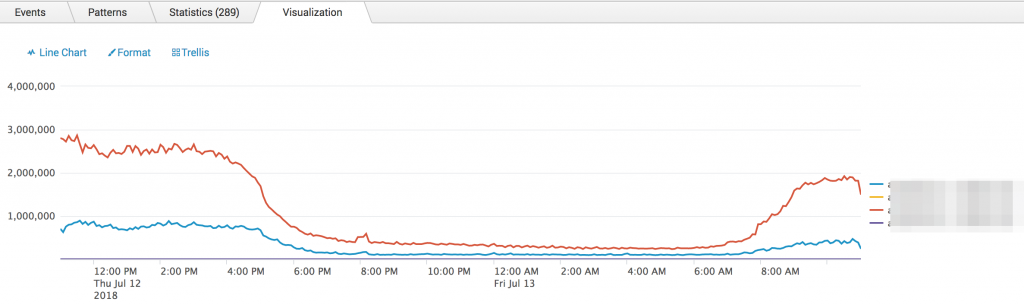# RHEL 7 UDP metrics into splunk metrics index

We were discussing this on splunk-usergroups slack, and I said I should post it here and vraptor and dawnrise urged me to do so quickly — so here I am.  (Thanks vraptor and dawnrise!)

First up, a script to use the nstat tool to grab some kernel UDP metrics and write them out in a format compatible with Splunk’s metrics store:

``````#!/bin/bash
FORMAT='"%s","%s","%s"\n'
typeset -A MAPPER
MAPPER=(
[UdpRcvbufErrors]="udp.buffer_errors"
)
populate_metrics() {
NOW=`date +%s`
printf \$FORMAT "metric_timestamp" "metric_name" "_value"
while read METRIC VALUE JUNK; do
printf \$FORMAT "\$NOW" "\${MAPPER[\$METRIC]}" "\$VALUE"
done <  <( nstat -z \${!MAPPER[@]} | egrep -v "^#" )
}
populate_metrics
``````

The relevant inputs.conf:

``````[script://./bin/udp_metrics.sh]
index = my_metrics
sourcetype = metrics_csv
interval = 60``````

A search that uses it:

``````| mstats span=5m sum(_value) as value where index=my_metrics metric_name=udp.packets_received by host
| xyseries _time host value``````

Obligatory picture: